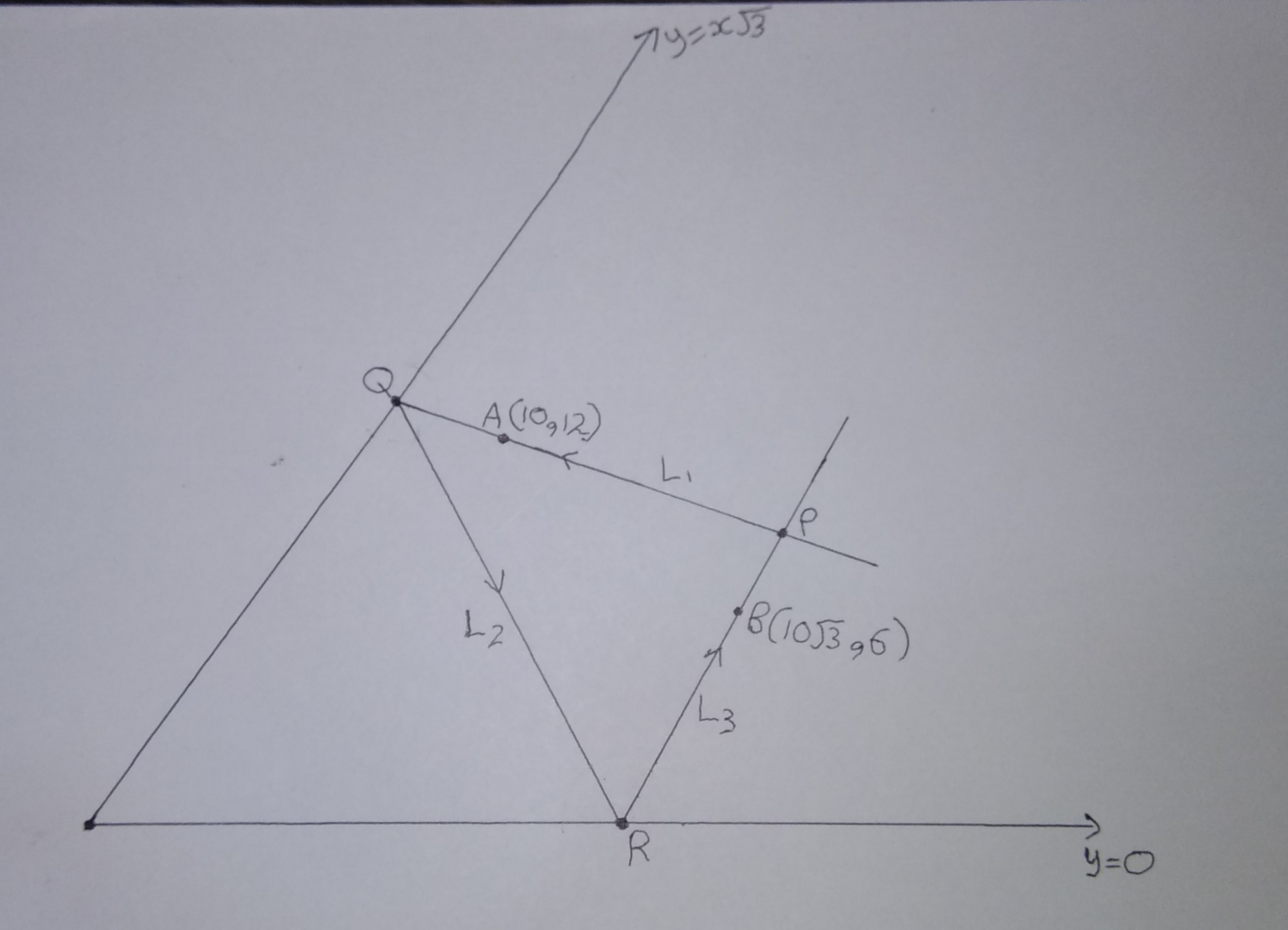# Triangle of Reflection

Geometry Level 3Passing through a point $A(10, 12)$, a line $L_1$ is incident on $y = x\sqrt3$ at point $Q(x_1, y_1)$. The reflected ray $L_2$ is incident on $y = 0$ at point $R(x_2, y_2)$. The now reflected ray $L_3$ passes through point $B(10\sqrt3, 6)$. Lines $L_1$ and $L_3$ meet at point $P(x_3 , y_3)$.

Find area of $\Delta PQR$. If area is of form $a\sqrt{b} \text{ where } a, b\in \N \text{ and } b \text{ is squarefree}$, enter answer as $a + b$.

All of my problems are original

×